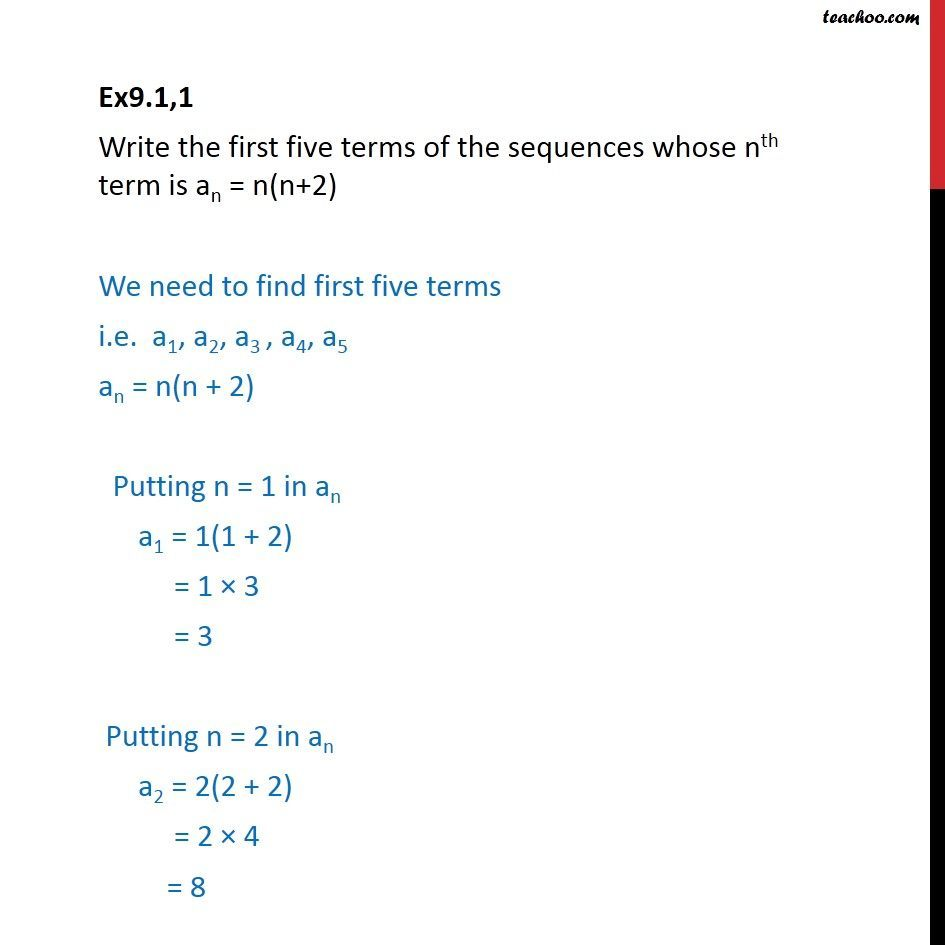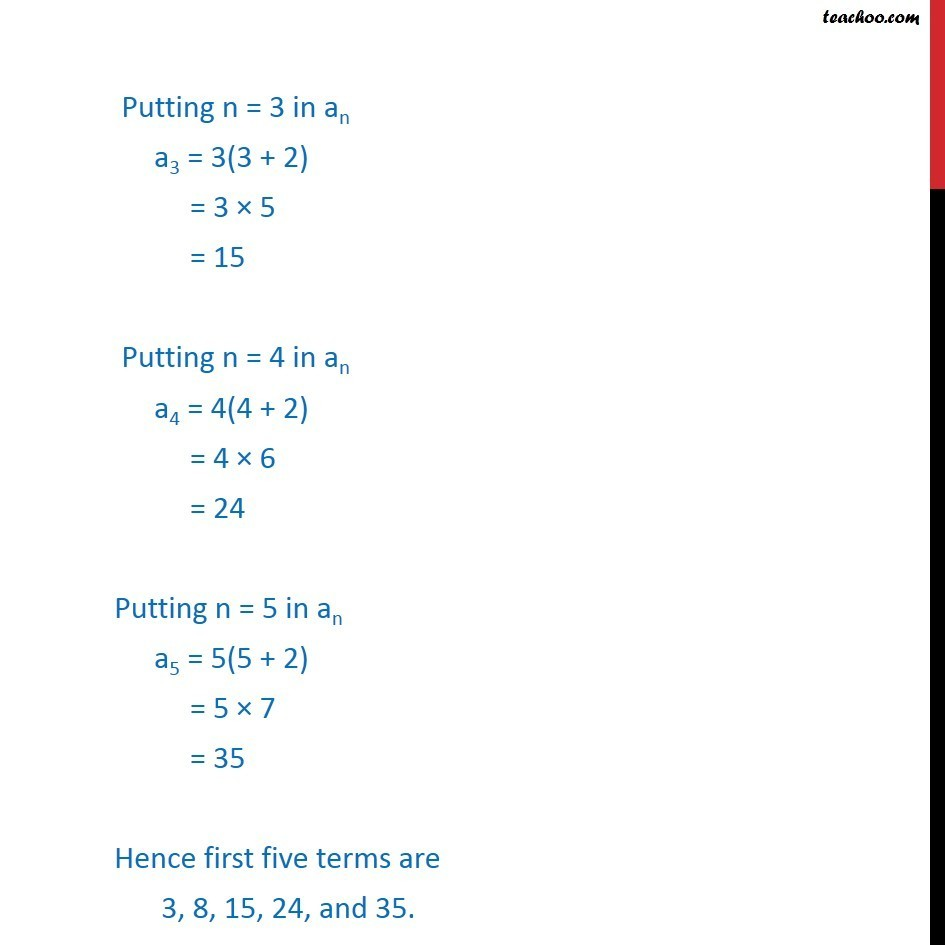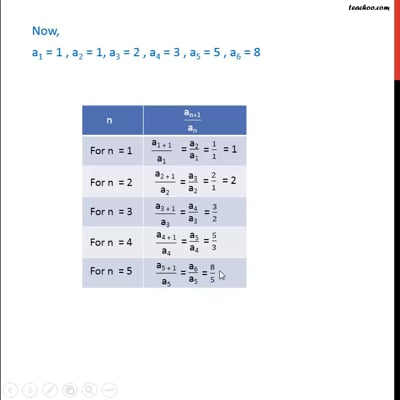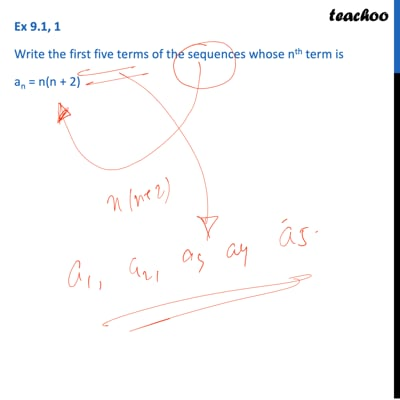Ex 8.1

Chapter 8 Class 11 Sequences and Series
Serial order wiseThis video is only available for Teachoo black usersThis video is only available for Teachoo black users

Learn in your speed, with individual attention - Teachoo Maths 1-on-1 Class

### Transcript

Ex9.1,1 Write the first five terms of the sequences whose nth term is an = n(n+2) We need to find first five terms i.e. a1, a2, a3 , a4, a5 an = n(n + 2) Putting n = 1 in an a1 = 1(1 + 2) = 1 × 3 = 3 Putting n = 2 in an a2 = 2(2 + 2) = 2 × 4 = 8 Putting n = 3 in an a3 = 3(3 + 2) = 3 × 5 = 15 Putting n = 4 in an a4 = 4(4 + 2) = 4 × 6 = 24 Putting n = 5 in an a5 = 5(5 + 2) = 5 × 7 = 35 Hence first five terms are 3, 8, 15, 24, and 35.